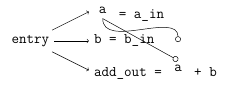# TikZ: using nodes inside nodes

I am trying to nest nodes like this:

\usemodule[tikz]
\usetikzlibrary{arrows, positioning}

\starttext
\starttikzpicture[font=\tt, remember picture]

\coordinate[above=1.25em of a-use.north] (c1);
\draw[-o] (a-def.south) edge[out=270,in=90] (c1) -- (a-use.north);

\stoptikzpicture
\stoptext


(I am using ConTeXt, but this should not be of relevance here.)

This produces:• Why is the path from one to the other a broken? It should go to the coordinate and proceed from there, not start over again.
• How can I align the two as with the baseline of the rest of the node's contents?

In LaTeX there is the tikzmark library, which offers a \subnode command. However, this library uses \newcommand and therefore throws an error with context. You can simulate the \subnode command as follows:

\def\subnode#1#2%
{\tikz[remember picture,baseline=(#1.base),inner sep=0pt,
outer sep=0pt,minimum width=0pt]\node(#1){#2};}


Setting the baseline aligns the a with the surrounding text. To remove the space introduced by the node, we take care that the node has the natural width of a by setting the surrounding space and the minimum width to 0pt. The only drawback of this is that now the anchors of the sub-nodes touch the contents, therefore we add 0.2ex below and above the sub-nodes when drawing the line.\usemodule[tikz]
\usetikzlibrary{arrows, positioning,calc}
\def\subnode#1#2%
{\tikz[remember picture,baseline=(#1.base),inner sep=0pt,
outer sep=0pt,minimum width=0pt]\node(#1){#2};}
\starttext
\starttikzpicture[font=\tt, remember picture]
\node[above=.1cm of add-b-in.north west, anchor=south west]
\node[below=.1cm of add-b-in.south west, anchor=north west]

\draw[-o] ($(a-def.south)+(0,-0.2ex)$) to[out=270,in=90]
($(a-use.north)+(0, 3.5ex)$) --
($(a-use.north)+(0, 0.2ex)$);
\stoptikzpicture
\stoptext


You can use subnode from tikzmark library to use part of a text as a node to be referenced.

\node[above=.1cm of add-b-in.north west, anchor=south west]


This solves the second question.

About the first question you are using edge which draw a line between two points, but don't move the origin, so

\draw[-o] (a-def.south) edge[out=270,in=90] (c1) -- (a-use.north);


draws a line between (a-def.south) and (c1) and a second between (a-def.south) and (a-use.north).

You want to use to[out=270,in=90] instead of edge.

\documentclass[tikz,border=2mm]{standalone}
\usetikzlibrary{positioning, arrows, tikzmark}

\begin{document}
\begin{tikzpicture}[remember picture]